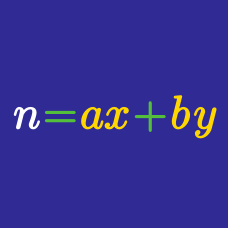Number Theory

# Integer Equations - Transformations

How many solutions are there to the inequality $3 when $x,y,z$ are non-negative integers?

How many solutions are there to the inequality $x+y+z\le6,$ where $x,y,z$ are non-negative integers?

How many solutions are there to the equation $a+b+c+d=15,$ where $a,b,c,d$ are integers such that $a\ge1, b\ge2, c\ge3, d\ge4?$

Amy is packing up for her camping trip. Her bag can currently fit up to ten bottles of drink. How many ways are there for her to pack her beverages including at least $4$ water bottles and at least $1$ bottle of coke?

Note: Her selection must consist of only water and coke.

How many solutions are there to the equation $x+y+z=16,$ when $x,y$ are positive odd numbers and $z$ is a positive even number?

×